# GSEB Class 6 Maths Notes Chapter 4 Basic Geometrical Ideas

This GSEB Class 6 Maths Notes Chapter 4 Basic Geometrical Ideas covers all the important topics and concepts as mentioned in the chapter.

## Basic Geometrical Ideas Class 6 GSEB Notes

Introduction:
Geometry has a long and rich history. The word Geometry has been derived from the Greek word ‘Geometron’, ‘Geo’ means Earth and ‘metron’ means measurement. Geometrical ideas are used in art, architecture, measurement, etc. We shall learn about basic elements point, line and plane.

Point:

• A small dot marked by a sharped end of a pencil on a sheet of paper or a tiny prick made by a fine needle or pin on a paper, Bindi are examples of a point.
• A point is denoted by a single capital letter like A, B, C.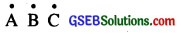These will be read as point A, point B and point C.
A point determins a location. It depicts the exact position of an object. A point has no length, breadth or height. It does not have any size.Line Segment:
A line segment is a portion of a line or a straight path from A to B is called line segment.
A and B are two end points of segment AB.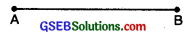There is a unique line segment joining two given points A and B. It is denoted by AB.

Notes :

• A line segment has two end points and a line has none.
• There is no difference between line segment AB and line segment BA.
• A line segment is completely known if its end points are known.

Remarks:
Sometimes we simply use the word ‘segment’ for a line segment.

Example: The line segment AB is simply written as segment AB.

Line:
A line is obtained when a line segment like AB is extended on both sides indefinitely. It is denoted by AB. It has no end point.Ray:
Part of a line having one end point and extending endlessely in one direction is called a ray. This end point is called the initial point of the ray.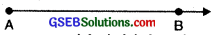AB is a ray with initial point A and extended indefinitely from A to B.

Parallel Lines:
The lines which .do not meet are said to be parallel lines.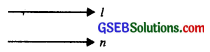Examples :

• The opposite edges of the ruler
• The cross bars of the window.

Parallel lines are shown in the above figure.

Plane:
A plane is a flat surface which extends indefinitely in all the directions. It has no boundary.

Intersecting Lines: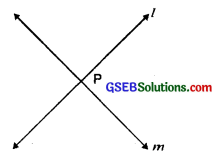If there is a point P common to two lines / and m, we say that the two lines intersect at the point P and this point P is called the point of intersection of the given lines.

Concurrent lines: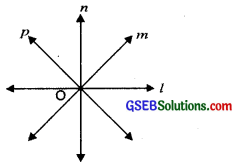Three or more lines in a plane when pass through a same point are called concurrent lines and that point is called the point of concurrence.

Lines l, m, n, p pass through O are called concurrent lines and O is called the point of concurrence.Collinear Points:
Three or more points in a plane are said to be collinear, if they all lie on the same line.Curves:
A set of points forming a continuous line is called a curve.
Generally ‘curve’ means not straight.
1. Simple curve. If a curve does not cross itself, then it is called simple curve.
For example :2. Open curve:
A curve in which the ends do not meet is called open curve.
For example :3. Closed curve:
A curve such as circular or Triangle. That a complete loop is called closed curve. Closed curve has no end points.
For example :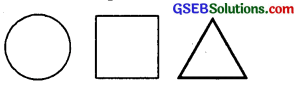Polygons:
Polygon means ‘Poly’ and ‘gon’. ‘Poly’ means ‘many’ and ‘gon’ means sides. ‘Polygon’ means ‘having many sides.’ A plane figure bounded by three or more line segment is called a polygon.

Notes :

• Any two sides with a common end point are called adjacent sides of polygon.
• The end points of the same side of the polygon are called adjacent vertices.
• The line joining the non-adjacent vertices are called diagonals of the polygon.
• A polygon is always a closed figure made up of only line segments. These line segments are called sides of the polygon.

Types of Polygons:
Polygons are further divided into various categories, depending upon the number of line segments they have
1. A three-sided polygon is called a Triangle.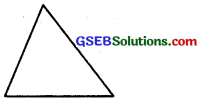2. A four-sided polygon is called a Quadrilateral.3. A five-sided polygon is called a Pentagon.4. A six-sided polygon is called a Hexagon.Similarly seven, eight, nine and ten sided polygons are called heptagon, octagon, nonagon and decagon respectively.

Regular Polygon:
If all sides of a polygon are equal and all angles are also equal, then it is called a regular polygon.Angle:
An angle is a figure formed by two rays with the same initial point.
The common initial point is called the vertex of the angle and the rays forming the angle are called its arms.

In the fig. O is the vertex and rays $$\overrightarrow{\mathrm{OA}}$$ and $$\overrightarrow{\mathrm{OB}}$$ are the turns of the angle.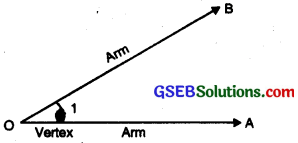Naming an Angle:
The angle shown is denoted by L\ or LO or Z.AOB or Z.BOA.
Remark:
Two line segments with a common end point also determine the angle at that point.

Interior and Exterior of an Angle
Let CAB be an angle. All points of the plane of the angle are divided into three parts.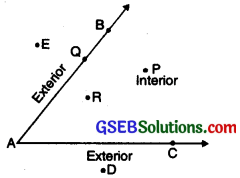• All interior points (such as P and R) of the angle i.e. those points which lie inside the angle. This part of the plane is called the interior of the angle.
• The part of the plane in which all exterior points (such as D and E) lie, is called the exterior of the angle.
• All points (such as Q) which lie on the angle itself is called the boundary of the angle.
Note: An angle separates its interior from its exterior.

Triangle:
A triangle is a closed figure made up of three line segments. Let A, B and C be three distinct non-collinear points. The figure formed by the three line segments AB, BC and CA by joining these non-collinear points A, B, C in pairs is called a Triangle. The symbol A is used to denote the word triangle. Thus, ∆ABC is read as ‘Triangle ABC’.

• The three points A, B and C are called the vertices of the triangle ABC.
• The three line segments AB, BC and AC are called the sides of the triangle.
• The three angles BAC, ABC and BCA are called the angles of the triangle ABC.The six parts, namely three sides and three angles, of a triangle taken together are called the six elements of the triangle. Two sides always include an angle and two angles always include a side. Each pair of two sides has a vertex in common.

Note: We may denote the three angles of a ∆ABC by the symbols ∠A and ∠B and ∠C which stand for the angles, ∠BAC, ∠ABC and ∠BCA respectively.

Side Opposite to the Vertex and Vertex Opposite to the Side
In fig. we observe that sides AB and AC meet at the vertex A. We say that BC is the side opposite vertex A, and A is the vertex opposite side BC.Similarly, CA is the side opposite vertex B and B is the vertex opposite side CA. AB is the side opposite vertex C, and C is the vertex opposite side AB.

Interior and Exterior of a Triangle:
A triangle divides all the points in a plane into three parts :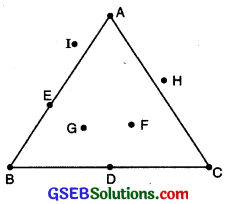• The part of the plane made by all those points that are enclosed by the triangle is called the interior of the triangle. In the figure, points F and G are in the interior of ∆ABC.
• The part of the plane made by the points that are on the triangle is called the boundary of the triangle. In the figure, A, E, B, D and C arc on the boundary of ∆ABC. The interior of ∆ABC together with its boundary is called the triangular region.
• The part of the plane made by the points that are not enclosed by the triangle is called the exterior of the triangle. In the figure H and I are in the exterior of ∆ABC.The word quadrilateral is derived from two words ‘Quadri’ and ‘lateral’. ‘Quadri’ means ‘four’ and ‘lateral’ means ‘side’. A closed figure with four line segments is called a quadrilateral. The quadrilateral has four sides, and four angles.
For example Square, Kites, Rectangles are all quadrilaterals.

A quadrilateral is named by writing its vertices in a cyclic order. This following quadrilateral ABCD.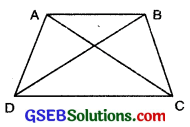• has four sides, namely AB, BC, CD and DA.
• has four vertices, namely A, B, C and D.
• has four angles, namely ∠A, ∠B, ∠C and ∠D.
• has two diagonals AC and BD.

In the figure AB, BC; BC, CD; CD, DA; DA, DA, AB are four pairs of adjacent sides of quadrilateral ABCD.

Opposite sides:
In the figure, AB, CD and AD, BC are two pairs of opposite sides of the quadrilateral ABCD.

In the figure, ∠A and ∠B, ∠B and ∠C, ∠C and ∠D, ∠D and ∠A are four pairs of adjacent quadrilateral ABCD.

Opposite angles:
In the figure, ∠A and ∠C, ∠B and ∠D are two pairs of opposite angles of quadrilateral ABCD.

Interior and Exterior of a Quadrilateral:
A quadrilateral divides all the points in a plane into three parts :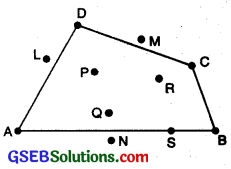Circle:
A circle is a closed curve all of whose points are equidistant from a fixed point.

Centre:
The fixed point is called the centre of the circle. In the figure, O is the centre of the circle.

The distance from the centre to any point on the circle is called radius of the circle.In the figure, OA, OB and OC are the radii of the circle. Also OA = OB = OC.

Diameter:
A line segment passing through the centre of the circle and whose end points lie on the circle is called diameter of the circle.
Diameter is twice the radius of circle
∴ d = 2r

Arc:
A part of circle is called the arc of a circle. $$\widehat{\mathrm{ACB}}$$ is the minor arc and $$\widehat{\mathrm{ADB}}$$ is the major arc.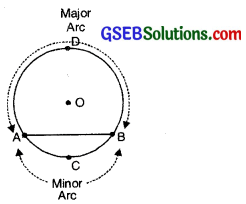Chord:
A line segment with its end points lying at the circle is called chord of the circle. In the above figure, AB is the chord.Segment:
A chord of a circle divides the circular region in the two parts, each part is called segment.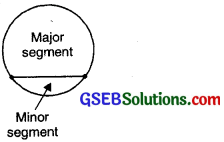Smaller part is called minor segment. The greatest part is called major segment.

Sector:
The area bounded by an arc and the two radii joining the end points of the arc with the centre is called a Sector.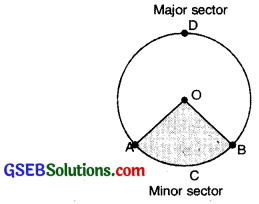In the figure, OACB is the minor sector and OADB is the major sector.

Interior and Exterior of a Quadrilateral
A circle divides all the points in the plane into three parts :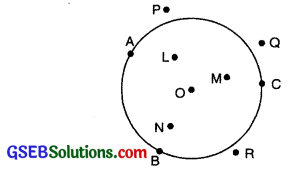• In the figure, points O, L, M, N are in the interior of circle.
• In the figure, points A, B, C are on the boundary of the circle.
• In the figure, points P, Q, R in the exterior of circle.